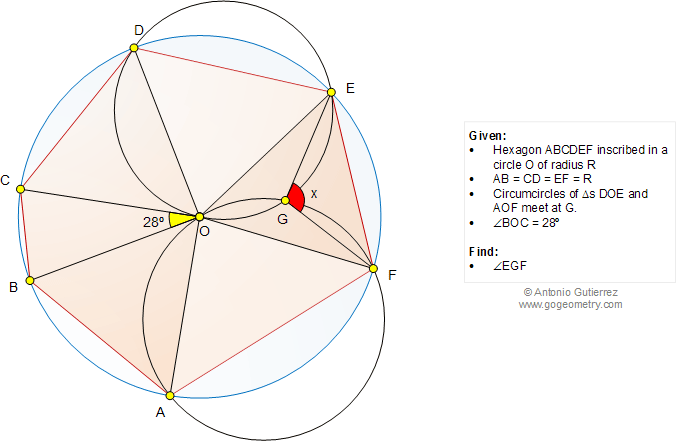# Online Math: Geometry Problem 1049: Hexagon inscribed, Circle, Circumcircle, Congruence, Angles, Polya's Mind Map. Level: School, College

< PREVIOUS PROBLEM  |  NEXT PROBLEM >

 The figure below shows a hexagon ABCDEF inscribed in a circle O of radius R so that AB = CD = EF = R. Circumcircles of triangles DOE and AOF meet at G. If the measure of angle BOC is 28 degrees, find the measure of angle EGF.Home | SearchGeometry | Problems | All Problems | Open Problems | Visual Index | 10 Problems | Problems Art Gallery Art | 1041-1050 | Circle | Hexagon | Circumcircle | Triangle | Angle | Congruence | Email | by Antonio Gutierrez

Add a solution to the problem 1049
Last updated: Oct 13, 2014